# Worksheet on Venn diagrams

Question 1
if U = {1,2,3,4,5,6,7,8,9,10}  , A ={1,2,3,4} , B={3,4,5,6}  and C ={2,3,6,7}
Show these on Venn diagram
a. $A - B$
b. $B - A$
c. $A \cap B \cap C$
d. $A \cup B \cup C$
e. $A \cup C$
f. $B \cup C$
Solution
Here is the Venn diagram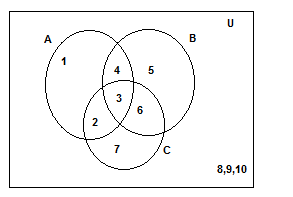a. A -B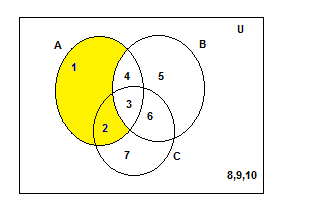A -B ={1,2}
b. B -A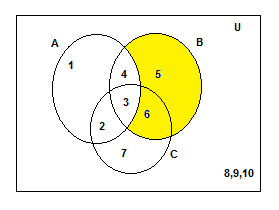B -A  ={5,6}
c. $A \cap B \cap C$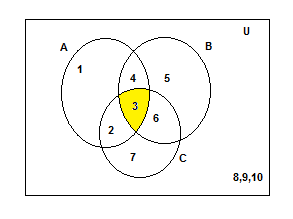$A \cap B \cap C$
={3}
d. $A \cup B \cup C$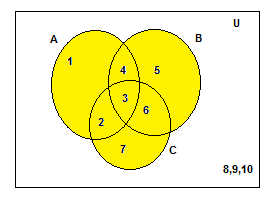$A \cup B \cup C$
=={1,2,3,4,5,6,7}
e. $A \cup C$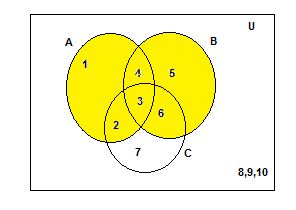$A \cup C$ ={1,2,3,4,5,6}
f. $B \cup C$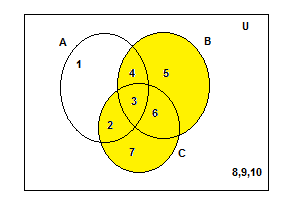$B \cup C$={2,3,4,5,6,7}

Question 2
Draw the Venn diagrams to illustrate the following relationship among sets E, M and U, where E is the set of students studying English in a school, M is the set of students studying Mathematics in the same school, U is the set of all students in that school.
(i) All the students who study Mathematics study English, but some students who study English do not study Mathematics.
(ii) There is no student who studies both Mathematics and English.
(iii) Some of the student’s study Mathematics but do not study English, some study English but do not study Mathematics, and some study both.
(iv) Not all students study Mathematics, but every student studying English studies Mathematics.
Solution
(i)
Since all the students who study mathematics study English, but some students who study English do not study Mathematics. Therefore, M ⊂ E ⊂ U Thus the Venn Diagram is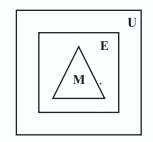(ii) Since there is no student who study both English and Mathematics Hence,
E ∩ M = φ.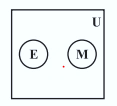(iii) Since there are some students who study both English and Mathematics, some English only and some Mathematics only.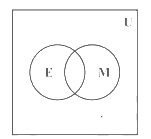(iv) Since every student studying English studies Mathematics. Hence, E ⊂ M ⊂ U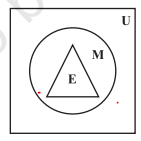Question 3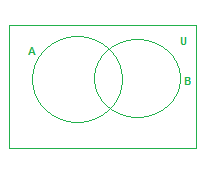a. $A^c \cap B^c$
b. $A \cap B^c$
c. $A^c \cap B$
Solution
a.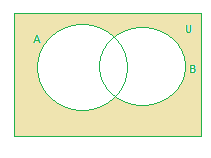b.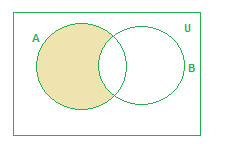c.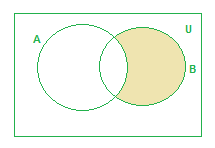Question 4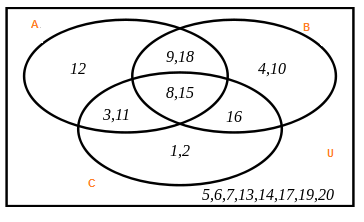Study the above Venn diagram and answer below questions
a.  $A \cap B \cap C$
b. $A \cap B$, $B \cap C$
c. $A^c \cap B$
d. $A \cup B \cup C$
e. $A^c \cap B^c \cap C^c$
Solution
a. {8,15}
b. {9,18,8,15}
{6,15,16}
c. {4,10,16}
d. {1,2,3,4,8,9,10,11,12,15,16,18}
e. {5,6,713,14,17,19,20}

Question 5
Study the below Venn diagram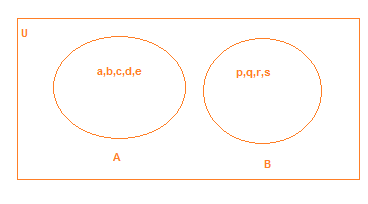(i)  A and B are disjoint sets. True or False
(ii) $A \cap B$ is an empty set. True or False
(iii) Find the value of $A \cup B$
Solution
(i) True
(ii) True
(iii) {a, b,c,d,e,p,q,r,s}

Question 6
Draw the Venn diagram
a. U = {x: x is a natural number and x < 20}
P = {1,2,3,4,5,6,7}
Q = {2,3,4}
b.  N = {x: x is a natural number}
W = {x: x is whole number}
U = {x: x is a set of real numbers}
c. U = {people who live in India};
V = {people who live in Uttar Pradesh}
W= {people who live in Lucknow}
X= {People who live in Hyderabad}
d.
X = Set of natural numbers,
Y = Set of prime numbers and
Z = Set of even prime numbers.